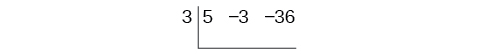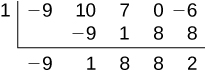# 5.4 Dividing polynomials  (Page 3/6)

 Page 3 / 6

## Using synthetic division to divide a second-degree polynomial

Use synthetic division to divide $\text{\hspace{0.17em}}5{x}^{2}-3x-36\text{\hspace{0.17em}}$ by $\text{\hspace{0.17em}}x-3.\text{\hspace{0.17em}}$

Begin by setting up the synthetic division. Write $\text{\hspace{0.17em}}k\text{\hspace{0.17em}}$ and the coefficients.Bring down the lead coefficient. Multiply the lead coefficient by $\text{\hspace{0.17em}}k.\text{\hspace{0.17em}}$Continue by adding the numbers in the second column. Multiply the resulting number by $\text{\hspace{0.17em}}k.\text{\hspace{0.17em}}$ Write the result in the next column. Then add the numbers in the third column.The result is $\text{\hspace{0.17em}}5x+12.\text{\hspace{0.17em}}$ The remainder is 0. So $\text{\hspace{0.17em}}x-3\text{\hspace{0.17em}}$ is a factor of the original polynomial.

## Using synthetic division to divide a third-degree polynomial

Use synthetic division to divide $\text{\hspace{0.17em}}4{x}^{3}+10{x}^{2}-6x-20\text{\hspace{0.17em}}$ by $\text{\hspace{0.17em}}x+2.\text{\hspace{0.17em}}$

The binomial divisor is $\text{\hspace{0.17em}}x+2\text{\hspace{0.17em}}$ so $\text{\hspace{0.17em}}k=-2.\text{\hspace{0.17em}}$ Add each column, multiply the result by –2, and repeat until the last column is reached.The result is $\text{\hspace{0.17em}}4{x}^{2}+2x-10.\text{\hspace{0.17em}}$ The remainder is 0. Thus, $\text{\hspace{0.17em}}x+2\text{\hspace{0.17em}}$ is a factor of $\text{\hspace{0.17em}}4{x}^{3}+10{x}^{2}-6x-20.\text{\hspace{0.17em}}$

## Using synthetic division to divide a fourth-degree polynomial

Use synthetic division to divide $\text{\hspace{0.17em}}-9{x}^{4}+10{x}^{3}+7{x}^{2}-6\text{\hspace{0.17em}}$ by $\text{\hspace{0.17em}}x-1.\text{\hspace{0.17em}}$

Notice there is no x -term. We will use a zero as the coefficient for that term.The result is $\text{\hspace{0.17em}}-9{x}^{3}+{x}^{2}+8x+8+\frac{2}{x-1}.$

Use synthetic division to divide $\text{\hspace{0.17em}}3{x}^{4}+18{x}^{3}-3x+40\text{\hspace{0.17em}}$ by $\text{\hspace{0.17em}}x+7.$

$3{x}^{3}-3{x}^{2}+21x-150+\frac{1,090}{x+7}$

## Using polynomial division to solve application problems

Polynomial division can be used to solve a variety of application problems involving expressions for area and volume. We looked at an application at the beginning of this section. Now we will solve that problem in the following example.

## Using polynomial division in an application problem

The volume of a rectangular solid is given by the polynomial $\text{\hspace{0.17em}}3{x}^{4}-3{x}^{3}-33{x}^{2}+54x.\text{\hspace{0.17em}}$ The length of the solid is given by $\text{\hspace{0.17em}}3x\text{\hspace{0.17em}}$ and the width is given by $\text{\hspace{0.17em}}x-2.\text{\hspace{0.17em}}$ Find the height, $\text{\hspace{0.17em}}t,$ of the solid.

There are a few ways to approach this problem. We need to divide the expression for the volume of the solid by the expressions for the length and width. Let us create a sketch as in [link] .

We can now write an equation by substituting the known values into the formula for the volume of a rectangular solid.

$\begin{array}{ccc}\hfill V& =& l\cdot w\cdot h\hfill \\ \hfill 3{x}^{4}-3{x}^{3}-33{x}^{2}+54x& =& 3x\cdot \left(x-2\right)\cdot h\hfill \end{array}$

To solve for $\text{\hspace{0.17em}}h,\text{\hspace{0.17em}}$ first divide both sides by $\text{\hspace{0.17em}}3x.$

$\begin{array}{ccc}\hfill \frac{3x\cdot \left(x-2\right)\cdot h}{3x}& =& \frac{3{x}^{4}-3{x}^{3}-33{x}^{2}+54x}{3x}\hfill \\ \hfill \left(x-2\right)h& =& {x}^{3}-{x}^{2}-11x+18\hfill \end{array}$

Now solve for $\text{\hspace{0.17em}}h\text{\hspace{0.17em}}$ using synthetic division.

$h=\frac{{x}^{3}-{x}^{2}-11x+18}{x-2}$The quotient is $\text{\hspace{0.17em}}{x}^{2}+x-9\text{\hspace{0.17em}}$ and the remainder is 0. The height of the solid is $\text{\hspace{0.17em}}{x}^{2}+x-9.$

The area of a rectangle is given by $\text{\hspace{0.17em}}3{x}^{3}+14{x}^{2}-23x+6.\text{\hspace{0.17em}}$ The width of the rectangle is given by $\text{\hspace{0.17em}}x+6.\text{\hspace{0.17em}}$ Find an expression for the length of the rectangle.

$3{x}^{2}-4x+1$

Access these online resources for additional instruction and practice with polynomial division.

## Key equations

 Division Algorithm

## Key concepts

• Polynomial long division can be used to divide a polynomial by any polynomial with equal or lower degree. See [link] and [link] .
• The Division Algorithm tells us that a polynomial dividend can be written as the product of the divisor and the quotient added to the remainder.
• Synthetic division is a shortcut that can be used to divide a polynomial by a binomial in the form $\text{\hspace{0.17em}}x-k.\text{\hspace{0.17em}}$ See [link] , [link] , and [link] .
• Polynomial division can be used to solve application problems, including area and volume. See [link] .

#### Questions & Answers

if sin15°=√p, express the following in terms of p
Norman Reply
prove sin²x+cos²x=3+cos4x
Kiddy Reply
the difference between two signed numbers is -8.if the minued is 5,what is the subtrahend
jeramie Reply
the difference between two signed numbers is -8.if the minuend is 5.what is the subtrahend
jeramie
what are odd numbers
micheal Reply
numbers that leave a remainder when divided by 2
Thorben
1,3,5,7,... 99,...867
Thorben
7%2=1, 679%2=1, 866245%2=1
Thorben
the third and the seventh terms of a G.P are 81 and 16, find the first and fifth terms.
Suleiman Reply
if a=3, b =4 and c=5 find the six trigonometric value sin
Martin Reply
ask
Ans
pls how do I factorize x⁴+x³-7x²-x+6=0
Gift Reply
in a function the input value is called
Rimsha Reply
how do I test for values on the number line
Modesta Reply
if a=4 b=4 then a+b=
Rimsha Reply
a+b+2ab
Kin
commulative principle
DIOSDADO
a+b= 4+4=8
Mimi
If a=4 and b=4 then we add the value of a and b i.e a+b=4+4=8.
Tariq
what are examples of natural number
sani Reply
an equation for the line that goes through the point (-1,12) and has a slope of 2,3
Katheryn Reply
3y=-9x+25
Ishaq
show that the set of natural numberdoes not from agroup with addition or multiplication butit forms aseni group with respect toaaddition as well as multiplication
Komal Reply
x^20+x^15+x^10+x^5/x^2+1
Urmila Reply
evaluate each algebraic expression. 2x+×_2 if ×=5
Sarch Reply

### Read also:

#### Get Jobilize Job Search Mobile App in your pocket Now!

Source:  OpenStax, Algebra and trigonometry. OpenStax CNX. Nov 14, 2016 Download for free at https://legacy.cnx.org/content/col11758/1.6
Google Play and the Google Play logo are trademarks of Google Inc.

Notification Switch

Would you like to follow the 'Algebra and trigonometry' conversation and receive update notifications?ByByBy OpenStaxBy OpenStaxBy OpenStaxBy OpenStaxBy Marion CabalfinBy Kimberly NicholsBy OpenStaxBy OpenStaxBy OpenStaxBy Laurence Bailen